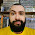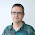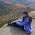## Sunday, February 19, 2012

### Colorize raster with GDAL python

As I was telling in my last post, what we usually want is to take a raster file, classify it, and output a png in a color scale.
The easiest way to do it, is using gdaldem with the color-relief option.
But is not in python.
So, if you want to integrate it in a script, or modify it's behavior easily, here you have a solution.
All the example data, including the whole script, dataset, etc, can be downloaded here.

The script:

The first thing to do is reading the color file. As in the gdaldem application, the file is plain text with four columns, value, red value, green value, blue value, and, optionally, the alpha value. We will consider alpha value to be 255 if is not set. The comments are indicated with the hash. This format is the one used in the GRASS r.colors utility
So a function is defined:

def readColorTable(color_file):
color_table = {}
if exists(color_file) is False:
raise Exception("Color file " + color_file + " does not exist")

fp = open(color_file, "r")
for line in fp:
if line.find('#') == -1 and line.find('/') == -1:
entry = line.split()
if len(entry) == 5:
alpha = int(entry)
else:
alpha=0
color_table[eval(entry)]=[int(entry),int(entry),int(entry),alpha]
fp.close()

return color_table


Once the table is read, is necessary to read the band in the raster file:

from os.path import exists
from osgeo import gdal
from osgeo.gdalconst import GA_ReadOnly
from struct import unpack

data_types ={'Byte':'B','UInt16':'H','Int16':'h','UInt32':'I','Int32':'i','Float32':'f','Float64':'d'}
if exists(raster_file) is False:
raise Exception('[Errno 2] No such file or directory: \'' + raster_file + '\'')

dataset = gdal.Open(raster_file, GA_ReadOnly )
if dataset == None:
raise Exception("Unable to read the data file")
band = dataset.GetRasterBand(raster_band)
values = band.ReadRaster( 0, 0, band.XSize, band.YSize, band.XSize, band.YSize, band.DataType )
values = unpack(data_types[gdal.GetDataTypeName(band.DataType)]*band.XSize*band.YSize,values)


Notice that the data types in the struct command and in the GDAL library haven't got the same names, so a dict has to be created to translate them. (Maybe there's a better way to do it, but this one works) A band has to be set. If the file has got only one band (as in our example), the value must be one. Then, two images must be created, one for the colors, an the other for the alpha channel:

import Image
import ImageDraw
base = Image.new( 'RGBA', (band.XSize,band.YSize) )
base_draw = ImageDraw.Draw(base)
alpha_mask = Image.new('L', (band.XSize,band.YSize), 255)


Now, the classification is done. There are two modes, the one that gives the exact color defined in the color file, and the one that calculates the color according to the value, and the two nearest colors in the palette. Here, to make more readable, only the exact color is shown:

import Image
classification_values = color_table.keys()
classification_values.sort()
for pos in range(len(values)):
y = pos/band.XSize
x = pos - y * band.XSize
for index in range(len(classification_values)):

if values[pos] <= classification_values[index] or index == len(classification_values)-1:
if index == 0:
index = 1
elif index == len(classification_values)-1 and values[pos] >= classification_values[index]:
index = index + 1
color = color_table[classification_values[index-1]]
base_draw.point((x,y), (color,color,color))
alpha_draw.point((x,y),color)

base_draw.point((x,y), (int(r),int(g),int(b)))
alpha_draw.point((x,y),int(a))

break


The first thing to do is getting the values of the color table. Then, for each pixel, the pixel position is calculated. To know the color value to be used, is necessary to iterate all the values in the color table to see which one is just over the pixel value. There is a special case if the pixel value is under the minimum value of the scale. Then, the pixel is set in the color image and the alpha channel. See the break statement to exit the loop.
Once the image and the alpha channel are calculated, the alpha channel has to be blend with the color channel before saving the result:

color_layer = Image.new('RGBA', base.size, (255, 255, 255, 0))
base = Image.composite(color_layer, base, alpha_mask)
base.save(out_file_name)


And that's all.
In the example, everything is packed in a function, and is possible to call it from the command line.
To calculate the color in the -nearest_color_entry mode, the code would be like this for each channel:

r = color_table[classification_values[index-1]] + (values[pos] - classification_values[index-1])*(color_table[classification_values[index]] - color_table[classification_values[index-1]])/(classification_values[index]-classification_values[index-1])


Of course, there is also the special case when the pixel value is lower than the lowest value in the scale or higher than the higher one. The solution is in the script.

Running the code:

We will use the same data of the last post (elevation data from the USGS).
All the sample data and the script is here.
A color file example could be this one (for our elevation data)

1600 166 122 59
1900 180 165 98
2200 188 189 123
2500 74 156 74
2800 123 189 83
3100 165 205 132
3300 255 255 255

To create the file, just type:
python colorize.py -exact_color_entry w001001.adf colorfile.clr out.png
or
python colorize.py -nearest_color_entry w001001.adf colorfile.clr out.png

Sample output with the -exact_color_entry option
Sample output with the default behaviour
It is possible to call the function from another python script just by typing

from colorize import raster2png
try:
raster2png(file_name,colorfile_name,out_file_name,band,discrete)
except Exception, ex:
print "Error: " + str(ex)


If discrete is True, the output is with the exact color in the palette. If is False, with the continuous mode.

1.The script not work...

1.Hello,
Have you tried with the original file or another one? Which error is giving?

2.Hi Roger,

I referred your codes to visualize my DEM file. I have a color table which generates a grayscale image. I want to create a color table that creates a color image. the classification values of the image are 0-255. Should I create a color table for 0-255, each number assigned with a RGB value ?
or should i write a new code to calculate the elevation of each pixel to assign a RGB value ?
kindly help me

1.No, you can create intervals. In your case, for instance:
0 166 122 59
50 180 165 98
100 188 189 123
150 74 156 74

would create a color every 50
Of course, if you really want to assign a color to each value, you can use a 255 colors scale

2.Thanks for replying Roger.
I eventually got a gray scale image using 255 color scale.
I tried creating color with every 100 and it doesn't seem to work.
here is my code for color mapping,

mapping = {}
with open(fname, 'r') as f:
for r in f.readlines():
in_vals = r.split(',')
in_val = [int(v) for v in in_vals]
mapping[in_val] = in_val[1:]
return mapping

steps = len(color_table.keys())

def get_normalized(orig_val, min_val, max_val, steps):
if orig_val < min_val:
raise ValueError("Invalid input val: below minimum value: %s" % orig_val)
if orig_val > max_val:
raise ValueError("Invalid input val: above maximum value: %s" % orig_val)

val_range = max_val - min_val
per_step = val_range/steps
above_min = orig_val - min_val
normalized_div = divmod(above_min, per_step)
if normalized_div:
return int(normalized_div +1)
else:
return int(normalized_div)

base_draw = Image.new('RGB', (band.XSize,band.YSize))
buff = ''
for pos, val in enumerate(values):
#if len(buff)<p.size:
# buff += val
# continue

#v = p.unpack(buff)
y = pos/band.XSize
x = pos - y * band.XSize
v = val

# this one is cruicial here: we get normalized
# input height value, within range from 0 to 255
try:
normalized_val = get_normalized(v, min_val, max_val, steps)
color = [normalized_val]*3
#color = color_table[normalized_val]
base_draw.putpixel((x,y), (color,color,color))
except ValueError:
# exception means value was invalid
pass
base_draw.show()

could you please let me know what's the problem ?